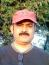# project selection

You are a project manager working on contract for a farming cooperative. They've decided to introduce six new wild juice drinks to the market that mix combinations of exotic juices to create some new exciting flavors. You've performed a feasibility study to determine the best way to go about producing, manufacturing, and marketing the wild juice drinks. Your research has yielded three alternatives that you want to compare based on today's value. The project's initial investment is \$29,000. Alternative A's cash flows are \$14,000 for year 1 and \$19,000 for year 2. Alternative B's cash inflows are \$21,000 for year 1 and \$20,000 for year 2. Alternative C's cash inflows are \$15,000 for year 1 and \$20,000 for year 2. Assume a 10 percent cost of capital. Which project should you recommend?

A)Alternative C

B)Alternative B

C)Alternative B and C are both acceptable

D)Alternative A### B? Project A(Net) CF(in) =

B?

Project A

(Net) CF(in) = 14/(1.10) + 19(1.10)^2

= 12.72727 + 22.99

= 35.71727

(Net) CF(out) = 29

NPV = 35.71727 - 29 = 6.71727

Project B

(Net) CF(in) = 21/(1.10) + 20(1.10)^2

= 19.0909 + 24.2

=43.2909

(Net) CF(out) = 29

NPV = 43.2909 - 29 = 14.2909

Project C

(Net) CF(in) = 15/(1.10) + 20(1.10)^2

= 13.636 + 24.2

=37.836

(Net) CF(out) = 29

NPV = 37.836 - 29 = 8.836

### endorse the calculations

endorse the calculations above and it is b

### I thought NPV calculation is

I thought NPV calculation is not part of the exam.

Can you pls explain the calculation here, I understood that we are calculating PV and adding it.

But didn't get why using 14, 19 instead of 14,000, 19,000 etc

pls explain,

### For simplicity and nothing

For simplicity and nothing else

B

### Can you explain as to why you

Can you explain as to why you have taken 14/1.1 for 1st year and 19* (1.10)^2 for the 2nd year

(Net) CF(in) = 14/(1.10) + 19(1.10)^2

Taking the formula PV=FV/[(1+r/100)]^n= 14 / [(1+10/100)]^1 is fine

Please explain the second part i.e., 19 * [(1+10/100)]^2

I have gone through this link, but not clear

http://pmi.books24x7.com/assetviewer.aspx?bookid=32027&chunkid=0192138754

B is the right answer### Project B,

based on the payback calculation done.

Regards

CN patil### PV calculation for inflow or Payback is appropriate here?

can we consider 10% cost of capital as rate of interest for PV calculation?

Cna anyone throw light ont this? I also got the right answer using the Payback period calcualtion.

Regards

CN Patil

### Yes, I think Iam wrong in

Yes, I think Iam wrong in taking 10% as rate of interest. Can you explain the payback period calculation or some reference based on which I can work out?### this how I did it...

Paybak for project A:
Investment =29,000
cash inflow=14000 (1 year)+19000 (2nd year)
First year: 29000-14000=15000
so, 15000/20000
=0.75
so it's 1year 9 months

Payback for project B
Investment=29,000
cash flow=21000+20000
First year: 29000-21000= 8000
so, 8000/ 20000
=0.4
so, it's 1year 4 months

Payback for projectC
Investment=29,000
cash flow=15000+20000
First year: 29000-15000= 14000
so, 14000/ 20000
=0.7
so, 1 Year 8 months

So, so it is project B based less number of months for payback.
*******************************************************************************************************

using ROI:

ROI
Project A
33000-29000/29000
0.13

Project B
41000-29000/29000
0.41

35000-29000/29000
0.20.

### Thank You very much

Thank You very much

### Answer

Payback for project B
Investment=29,000
cash flow=21000+20000
First year: 29000-21000= 8000
so, 8000/ 20000
=0.4
so, it's 1year 4 months
SEO for law firms